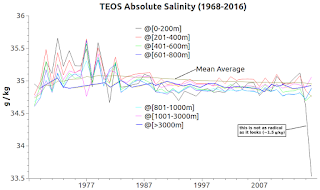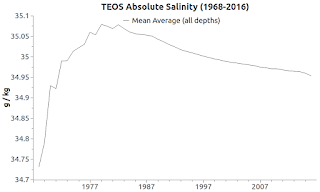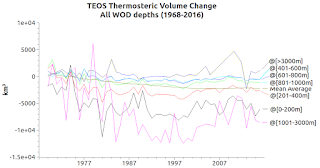## Saturday, October 14, 2017

### On Thermal Expansion & Thermal Contraction - 25Fig. 1a Conservative TemperatureFig. 1b CT Average
In the Thermodynamic Equation of SeaWater 2010 (TEOS-10) scheme of things, Conservative Temperature (CT), Absolute Salinity (SA), and Pressure (P) are key players.

CT is derived from in situ (measured) temperature taken at a given location using the function gsw_ct_from_t.

SA is derived from in situ (measured) salinity taken at a given location using the function gsw_sa_from_sp.

P is derived from measured depth at a given latitude using the function gsw_p_from_z.

The graph at Fig. 1a shows CT at the typical Dredd Blog "seven depth levels" (depth is 'height' in TEOS parlance), with one of the graph lines being the median (mean average).Fig. 2a Absolute SalinityFig. 2b SA Average

The graph at Fig. 1b shows the average CT of those seven depth levels.

In like fashion, the graphs at Fig. 2a and Fig. 2b show the same for SA.Fig. 3a Thermal Expansion / ContractionFig. 3b Thermal Expansion / Contraction Average

Since P is a function of depth, the pressure is derived from the depth of each CT and SA  in situ measurement.

The big story in today's post is the calculation of changes in thermal expansion and contraction at each of those depth levels.

The graph at Fig. 3a shows the changes in thermosteric volume at the seven depth levels, including a mean average of all seven combined.

The graph at Fig. 3b shows the average of those thermosteric volume changes.

The span of time involved begins in 1968 and ends in 2016, using all WOD measurements from all zones.

That span of time is due to my use of CTD and PFL datasets of the World Ocean Database (WOD).

I don't use the data acquired by older methods of gathering in situ measurements, preferring the more advanced gathering technology.

About half a century of data collection is recorded in the CTD and PFL datasets (about a billion temperature, salinity, and depth measurements).

As you can see in Fig. 3a and Fig. 3b, the results are not intuitive.

The results depart from the current thinking that thermal expansion has been the main source of sea level rise for a century.

The net result for the 1968-2016 span of time is a net -2171.6305236 km3 thermal contraction (not thermal expansion).

That value equates to about a 6 mm [~1/4 inch] thermosteric volume decrease (-2171.6305236 ÷ 361.841 = −6.001615416 mm [-0.236284071 inch]) ...  (the 361.841 value is the number of cubic kilometers necessary to raise the global mean average sea level by one millimeter).

Regular readers know that I have been experimenting to find a better way to calculate these values, and at this point I think using the seven ocean depth levels method is working well.

I have used the seven depth levels method with in situ temperature and salinity for quite a while.

The formulas for deriving the calculations were shown previously, along with the actual source code that does it (The Art of Making Thermal Expansion Graphs).

One difference in today's work is that the ten temperatures used in that linked-to example ("5.5,6.5,7.5,8.0,8.5,8.0,7.5,7.0,6.5,6.0, 5.5") are replaced with about a billion measured values that are used to produce the graphs shown in today's post.

What is most important in this calculation sequence (Fig. 3a) is that the individual depth levels' mass-volume must first be determined before calculating the thermal expansion coefficient.

That is because the seven depth levels don't all have the same mass-volume. (e.g. the 0-200 m level is different from the 1001-3000 m level in terms of mass-volume).

Notice in Fig. 3a that the depth level with the most thermal expansion is the >3000 m level (non-intuituve).

All the heat going into the oceans tends to move from warmer water to colder water over time.

As the heat works its way through the ocean basins, it causes expansion and contraction individual to that basin and the particular depth level.

BTW, the eustatic (non-thermal) sea level change graphs, measured by tide gauge stations featured in today's graphs, are posted here.

The next post in this series is here, the previous post in this series is here.

The ice is melting ... (Dr. Eric Rignot) ...